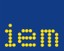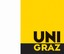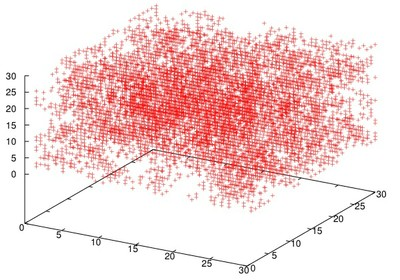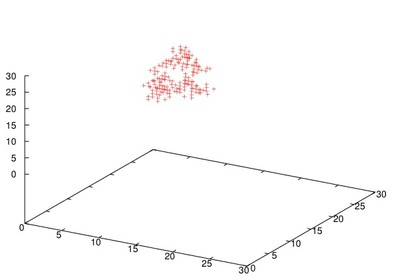##### Personal tools
You are here: Home CENTER - Clusters# CENTER Clusters in QCD

QCD (Quantum Chromo Dynamics) is the theory of quarks an gluons. Under normal conditions quarks are confined in bound states such as protons and neutrons, the main building blocks of ordinary matter. At very high temperatures, such as the ones shortly after the Big Bang, quarks can escape their bound states and become deconfined. The transition from confinement to deconfinement is a phase transition, vaguely similar to e.g. the boiling of water. Understanding the phenomenon of confinement and the deconfinement transition are important open questions in QCD.In the current project we analyze spatial structures in the behavior of quark sources. These structures are related to the so-called center of the gauge group, which somewhat simplified consists of three numbers -1,0,+1, which may be assigned to every point in space. Here space is discretized, i.e., it is a grid, where every coordinate x,y and z can assume the values 0,1, ... 39, and we use periodic boundary conditions. To every point (x,y,z) a number is assigned which may assume the values -1,0,1 or 2. The first three values are the center elements mentioned above, the number 2 is used for lattice points which were cut in a filtering process and are not taken into account in the analysis. The data files I provide have 40x40x40 lines, and in each line an integer number (-1,0,+1,2) is given for the corresponding grid point. A small C++ program which I provide reads in the data and puts them in an array with three coordinates.

An interesting observation is, that equal center values tend to form clusters in space: If two neighboring lattice points have the same center value (-1,0 or 1) then we put them in the same cluster. These clusters were identified and studied. Below the deconfinement temperature we find that the clusters have a size which is essentially independent of the temperature. At the critical deconfinement temperature the clusters start to grow, and one of them becomes infinitely large, i.e., it occupies a fixed fraction of the lattice (the clusters "percolates"). A simple physical argument can be given, that the percolation of the cluster is related to the deconfinement of quarks.

Questions I am interested in: Can one sonify the clusters? Can one distinguish the low and high temperature phases acoustically? Can one hear the transition into the deconfinement phase?

##### Document Actions